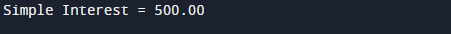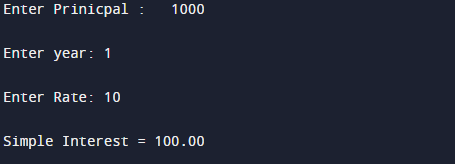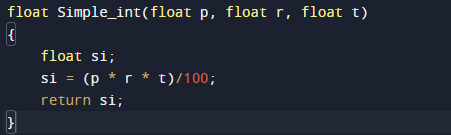# c program to calculate simple interest using function

In this tutorial you will learn about the c program to calculate simple interest using function and its application with practical example.

In this tutorial, we will learn to create a C program that will Calculate Simple Interest using function in C programming.

## Prerequisites

Before starting with this tutorial we assume that you are best aware of the following C programming topics:

• C Operators.
• C functions,
• basic input/output.

## What Is Simple interest?

Simple interest is basically a calculation of the interest charged on a given principal amount with in a particular part of time by the bank to customer or taken by bank.

Simple Interest = (p * n * r) / 100

here,    p = Principal,

n = Time Period or year.

r = Rate of Interest.

## Example

Let take an example of this,

p=5000,r=10,and t=1 year.

then,## Output## Algorithm

1. Firstly define Principal, rate and Time of loans.
2. Apply the formula.
3. Output Simple Interest.

## C Program to Calculate  Simple Interest.

First of all  we take principal ,rate and time  and pass them to function as a argument that will calculate the simple interest.let see a program for these.

## OutputIn the above program, we have first declared and initialized a set variables required in the program.

• a = for taking value of Principal.
• b = for taking value of rate.
• c= for taking value of time.
• p= for holding value of a.
• r = for holding value of b.
• t= for holding value of c.
• si= calculate simple interest.

This program takes three  values of principal,rate and time in a,b,c from user and then passing these value in function Simple_int(a,b,c) as argument the above function after  calculating value function returns simple interest.As shown in above figure. The value return by the function is hold by result variable and with help of result we can print the simple interest using function.

In this tutorial we have learn about the c program to calculate simple interest using function and its application with practical example. I hope you will like this tutorial.